﻿ Improve Diversity with OFDM Technique in V-BLAST Architecture
Journal of Telecommunications System & Management
• Research Article
• J Telecommun Syst Manage 2016, Vol 5(1): 121
• DOI: 10.4172/2167-0919.1000121

# Improve Diversity with OFDM Technique in V-BLAST Architecture

Kuldeep Shukla* and Nidhi Bhatt
*Corresponding Author: Kuldeep Shukla, Department of Electronics and Communication, SAL-ITER, Sola Bhadaj Road, Ahmedabad, India, Tel: 07965229333, Email: [email protected]

Received Date: Oct 27, 2015 / Accepted Date: Oct 30, 2015 / Published Date: Jan 03, 2016

### Abstract

There are several technologies to transmit any signal in wireless communication. Technologies are: Integration, Differentiation, TDM (Time Division Multiplexing), FDM (Frequency Division Multiplexing), OFDM (Orthogonal Frequency Division Multiplexing). In which OFDM is superior to any other technology. It is widely used in MIMO schemes also where multiple transmission and reception process done with different types of antennas. V-BLAST is the technique where the completion of strongest signal capturing and it gives sturdiest output with the help of different receiver approximation techniques like MMSE (Minimum Mean Square Error), ZF (Zero Forcing) and ML (Maximum Likelihood). In this paper we throw some light on why choose MMSE, ZF and ML to identify overall Throughput, adjustment in pre-coding, performance of FFT (Fast Fourier Transform) and IFFT (Inverse FFT) in OFDM-VBLAST. Also examine which receiver technique is widely and very useful in practical scenario.

Keywords: OFDM; MSE; Throughput; Pre-coding; FFT; ZF; MMSE; ML

#### Introduction

When any signal passed through air it has list numbers of interferences where core interference is ISI (Inter Symbol Interference). Integration applied on multipath air signal means it combine small number of signal division with fixed length assumption. Means integration is applied on time invariant signals but multipath air signals are always time variant. So, it is not applicable for wireless communication. Differentiation means large signal is divided into small number of signals with fix length. To process of total differentiation length of that signal should be fixed. That is why; it is not applicable to find-out the solution of wireless scenario .

TDM technique is fulfilling the criteria of wireless signaling. In general TDM means band-width is allocated to multiple users with division of time. It means TDM provide full bandwidth for small number of time to multiple users. But wireless signals take full time to transmit and receive the digital information. So, TDM technique is not fulfilling all the requirements. FDM technique delivers small bandwidth for full time. But the problem is if numbers of users are more than the division of that small band-width is very small and if those small band-width signal transmissions changes its phase in air than error output will captured. Whenever signal change its phase vector also shift its position to its original co-ordinates. When vector shifts than it produce disturbance on other user channel. It creates timing disturbances.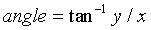(1)

This is nothing but process of aliasing at side of receiver with digital multipath signals. Another drawback is those digital signals are finite in time (time limited) but infinite in band (band unlimited) so ideal receiver cannot give adequate output. Solution of above drawback is orthogonally (OFDM) signal transmission. Orthogonal FDM deliver better algorithm compare with all other previous technology [2,3].

Orthogonal signal means projection of output vector in two vectors with minimum angle. Spectral is also orthogonal where dot product of those two vector is zero. That means projection is zero and gives largest hemming distance (Figure 1).

#### OFDM Mathematics

MSE in OFDM

If any function f (t) filtered under ag (t) filter where “a” is the weighted co-efficient of that filter for t1 to t2 time then, (Figure 2) Signal approximation f(t) by g(t); f(t) ≈ ag(t) over (t1, t2) and error is: e(t) = f(t)-ag(t).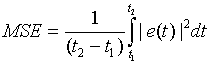(2)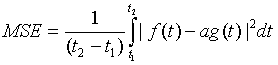(3)

MSE minimum at partial differenciation w.r.t. “a” when,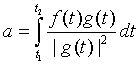(4)

This is orthogonal condition for minimum MSE where two vectors A and B are orthogonl and gives minimum error e(t) because of magnitude of A and B is equal with appropriate phase angle θ .

Throughput relation

As we seen before auto co-variance, integrator and differentiator is not used in transmitter.

Let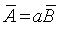or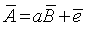(Pythagoras)

But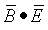or orthogonal so;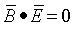then,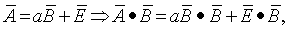“a” is optimally chosen then,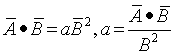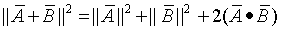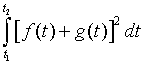In signals;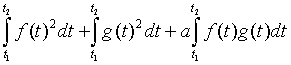=

Total Energy = Individual Energy + 2(Cross Energy)

For orthogonal cross energy is zero than through put becomes high.

Let’s take more than one gain with its respective weighted coefficient than, ag (t) = a1g1(t) + a2g2(t); where a1 and a2 are weighted co-efficient with optimal value of gain g1(t) and g2(t).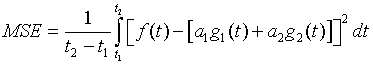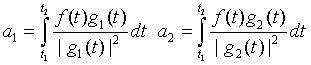The system is orthogonal so a1 and a2 are adjusting value and individual each other.

Motive of FFT in OFDM

If multiplication of total gain with its weighted code is taking more than one separate gain and weighted summation then,

ag (t) = a1g1(t) + a2g2(t);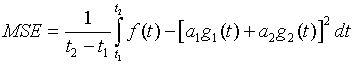(5)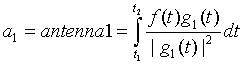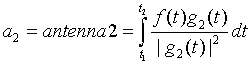Orthogonal that is why a1 and a2 are adjustable values and individual with each other. So, it is solved by gain adjusted pre-coding.

V-BLAST architecture: There are four BLAST technologies like D-BLAST, V-BLAST, H-BLAST and T-BLAST. D is known as Diagonal BLAST same as V for Virtual, H for Hybrid and T for Turbo. In these four technologies V-BLAST is very important technology. D-BLAST gives output but it is diagonally BLAST means Diagonal matrix have maximum energy (minimum error). V-VLAST has virtually division so it reduces time and increase accuracy over large bandwidth. H-BLAST and T-BLAST are faster than V-BLAST but suitable work for narrow bandwidth only [4-6].

Result analysis:

Probability where random signal: If < 0.5 than Zero otherwise One.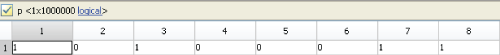Convert 0 and 1 to -1 and 1: Polar Signal ConversionThe combination of 2 × 2 antennas

sHat1 (2 × 2 Tx-Rx antenna):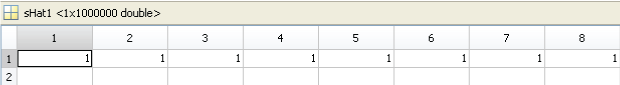sHat2 (2 × 2 Tx-Rx antenna):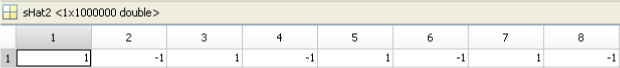sHat3 (2 × 2 Tx-Rx antenna):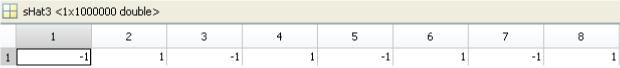sHat4 (2 × 2 Tx-Rx antenna):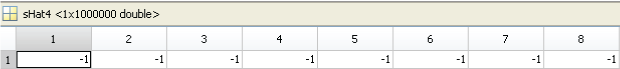Vector analysis: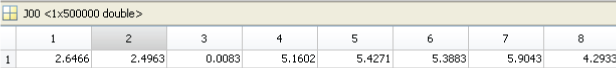J01 vector for MMSE (2 × 2 Tx-Rx antenna):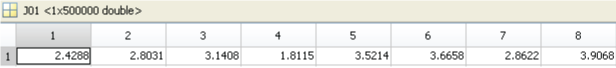J10 vector for MMSE (2 × 2 Tx-Rx antenna):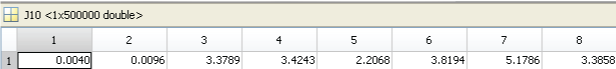J11 vector for MMSE (2 × 2 Tx-Rx antenna):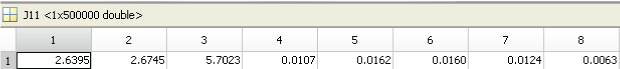Rvec (Received Vector) takes all the data from its individual vector J00, J01, J10, J11.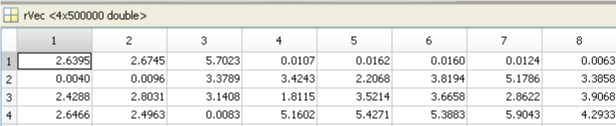Dd (Destination decimal Value-Which Antenna have Minimum Error Signal)“U” identifies which vector has a lowest signal error: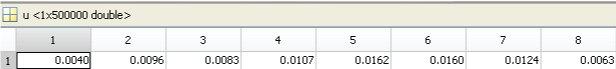nErr (n no. of Error where n is user defined):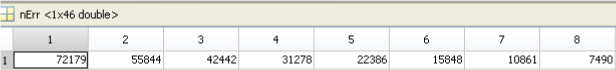Ber3=nErr/N (Ex: 72179/1000000=0.072179):-ML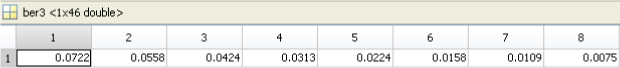Same as ber 1-MMSE: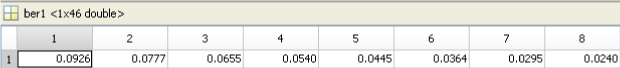And Ber2-ZF: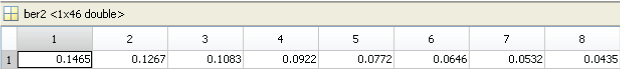For any random input signal threshold (or cutoff) is identified. In this paper cutoff is 0.5. Means for any random input signal if the value is higher than 0.5 outputs is 1 otherwise 0. Polar form conversion is used because check signal parameters in three dimention and gives the values -1 and 1 (Table 1 and Figures 3 and 4).

Parameter Value
No. of Transmitter 2
Overall Combination 4
SNR 0 to 55 dB
Block size 1000000
Type QPSK
Transmission Type QPSK with IFFT
Receiver Type V-BLAST with ML,ZF, MMSE

Table 1: Parameters overview.

This signal goes to 2 × 2 antenna (Means Two transmitters and Two receivers). So, It has four different combinations. By performing above blocks signal is in frequency domain, to idenify how much amount of noise will be added in signal convert signal to time domain by using IFFT. Receiver antennas received noisy signals and send to the FFT. FFT converts time domain signals to frequency domain for easy identification of noise. Now, “Rvec” collect and identify which vector has less amount of noise by which combination. “U” identify the amount of error. After this act techniques will come for point analysis of BER and SNR [7,8].

#### Conclusion

A purpose of this paper is to analyze and compare to identify the behavior of different receiver techniques. Practical performance using Mat-Lab gives you the disturbance in signal due to the noise at receiver.

By performing above parameters disturbance in ML (Minimum Likelihood) is in 10-4.9 BER and 18 dB SNR. But for smaller SNR values ML gives sharper output (Less BER) compare with MMSE and ZF.

In MMSE (Minimum Mean Square Error) disturbance is in 10-4.3 BER and 32 dB SNR. When perform with high SNR with less fluctuation MMSE is used.

In ZF (Zero Force) disturbance is in 10-3.9 BER and 32 dB SNR. When perform with large range of SNR ZF is used.

#### Acknowledgement

I would like to thank to Miss. Hetal N. Rao and Prof. Kiran Parmar. I also thank to my almighty.

#### References

Citation: Shukla K, Bhatt N (2015) Improve Diversity with OFDM Technique in V-BLAST Architecture. J Telecommun Syst Manage 4: 121. Doi: 10.4172/2167-0919.1000121

Copyright: ©2015 Shukla K, et al. This is an open-access article distributed under the terms of the Creative Commons Attribution License, which permits unrestricted use, distribution, and reproduction in any medium, provided the original author and source are credited.

Select your language of interest to view the total content in your interested language

##### Recommended Journals
Viewmore
###### Article Usage
• Total views: 9046
• [From(publication date): 3-2016 - Dec 15, 2019]
• Breakdown by view type
• HTML page views: 8929Can't read the image? click here to refresh